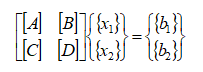Intel® oneAPI Math Kernel Library
Ask questions and share information with other developers who use Intel® Math Kernel Library.
6677 Discussions

## Avoid matrix inversion is it always possible?Beginner
153 Views

We need to solve a linear system with a dense matrix of complex numbers.

When the size of the matrix is lower than 4 GB (Microsoft limits for 64 bit systems) we can use MKL routines directly to solve:

{x} = {b}

Instead when the matrix is bigger than 4 GB we divide the matrix in blocks:The linear system becomes:From (1) we obtain:Solving equation (6) the solution x2 is obtained.

Using x2 in (3) it is easy to calculate x1.

This algorithm is interesting but introduces a matrix inversion.

This is a big problem when the linear system is ill conditioned.

Any suggestion to solve the problem avoiding the matrix inversion?

Thankyou

Gianluca